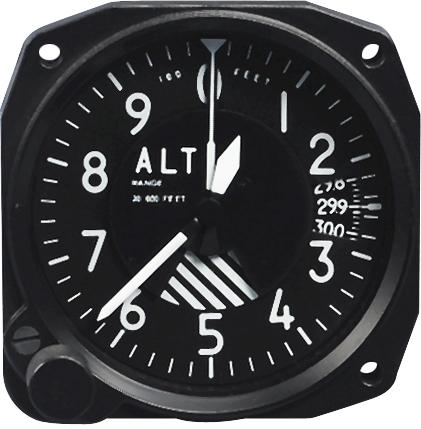# Altimeter

## Basic introduction

Altimeter: 　ɡāodùjì

The altimeter is a high-precision instrument with various ranges designed for many industrial applications and testing institutions.

Widely suitable for a variety of applications, its applications include precision workpiece inspection, multi-point inspection, measurement equipment monitoring and position measurement and many other fields.## Structural principle

The instrument includes a high-precision grating device, a measuring rod device with a dense bead guide rail, and high-performance high-speed sampling.

The altimeter uses a high-precision grating as the measurement reference, and an incremental grating ruler with a glass substrate is selected, so the measurement supports a large measurement range, is insensitive to vibration and impact, and has a definite temperature characteristic.

The scanning method of incremental grating ruler is photoelectric scanning, so there is no mechanical contact or wear. The electronic device subdivides the output signal to a very small measurement step of nanometer level, so as to ensure a small position error within a signal period.

## Barometric altimeter

The barometric altimeter is placed in the aircraft during the detection of aerial objects. It uses the relationship between air pressure and altitude to measure the altitude of the aircraft flight (also known as absolute altitude). ) Of the instrument.

Everyone knows that the pressure in the water is only determined by the depth of the water, P=ρgh. Atmospheric pressure is similar to this, which is produced by the gravity of the air on the surface. As the altitude increases, the thickness of the air on the surface decreases and the air pressure decreases. Therefore, the altitude value can be obtained by measuring the atmospheric pressure at the location and comparing it with the standard value. This is the basic working principle of the barometric altimeter. Suppose the atmospheric pressure at sea level is P0 and the local atmospheric pressure is P, then the altitude h=(P0-P)/(ρ*g). As the altitude increases, the temperature and pressure of the atmosphere gradually decrease, resulting in a decrease in density. A formula that does not consider this point is of no practical value.

Assuming that the density decreases uniformly with height, h=0 at sea level, ρ=ρ0, h=r (atmospheric thickness) at the outer boundary of the atmosphere, ρ=0, so ρ=ρ0 (h0-h )/H0, then the atmospheric pressure at altitude h is the sum of the atmospheric mass from h0 to h. Because it is a linear relationship, the atmospheric pressure at altitude h can be calculated as P(h)=ρ0 with the knowledge of the arithmetic sequence (h0-h)^2/(2h0), and the standard atmospheric pressure P0 at sea level and the air density ρ0 are both known, take P0=101kPa, and the air density ρ0=1.2kg/m^3, which can be used to calculate h0 =8400 meters, so the expression for altitude should be corrected to h=h0-sqrt(P/P0).

In fact, the thickness of the gas that contributes to the surface atmospheric pressure is indeed only on the order of tens of kilometers. More precisely, 99% of the atmospheric mass is concentrated within 30km of the surface, of which 50% is within 5.6 kilometers. %. Although the upper atmosphere above 100km has an important impact on the earth's environment, its density is already quite low.

Of course, the assumption of this linear relationship is only a rough approximation. It can be concluded from the hydrostatic equilibrium condition that the atmospheric density decreases exponentially with the increase in altitude. However, this sentence It is approximately true only when the atmosphere is statically stable. On this basis, NASA gives an empirical formula for near-Earth atmospheric temperature and pressure. All barometric altimeters use machinery or circuits to reproduce the correspondence between pressure and altitude. , But the difference in air density caused by climate change is completely impossible to estimate. This is a common problem with this type of altimeter. Therefore, it is better to use GPS based on solid geometry when highly accurate values ​​are required.

Related Articles